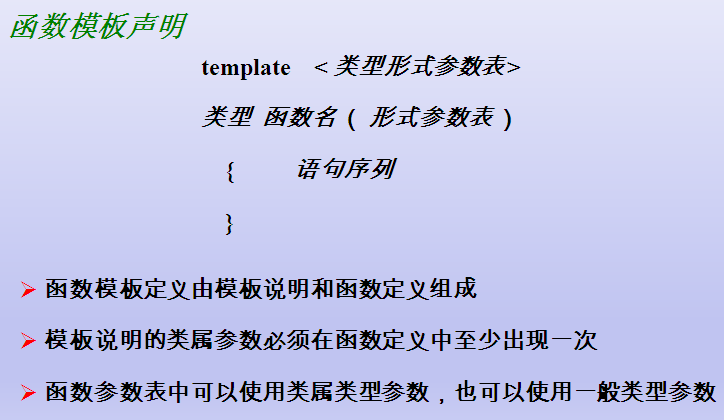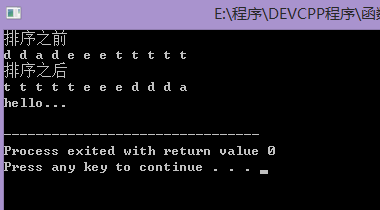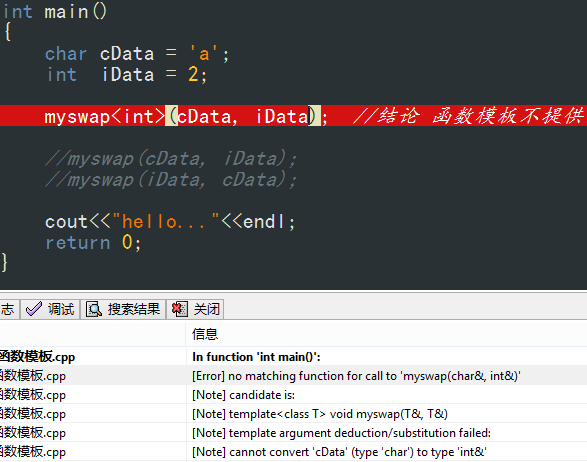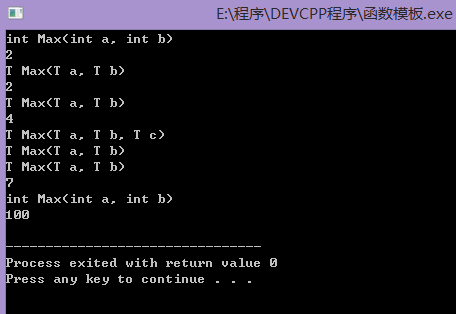C++函数模板及实现原理

C++为我们提供了函数模板机制。所谓函数模板，实际上是建立一个通用函数，其函数类型和形参类型不具体指定，用一个虚拟的类型来代表。这个通用函数就称为函数模板。

凡是函数体相同的函数都可以用这个模板来代替，不必定义多个函数，只需在模板中定义一次即可。在调用函数时系统会根据实参的类型来取代模板中的虚拟类型，从而实现了不同函数的功能。

为什么要有函数模板

下面，我们就通过一个例子来说明为什么需要有函数模板。

需求：写n个函数，交换char类型、int类型、double类型变量的值。

如果不适用函数模板的话，我们的代码需要这样写：

void swap(int &a, int &b)
{
int t = a;
a = b;
b = t;
}

void swap(char &a, char &b)
{
char t = a;
a = b;
b = t;
}
这样的代码总是很麻烦，几乎一样的代码却要重复写很多次，因为，我们就出现了函数模板机制。有了函数模板之后，我们的代码可以这样写：

#include<iostream>
using namespace std;

//template 关键字告诉C++编译器 我要开始泛型了.你不要随便报错
//数据类型T 参数化数据类型
template <typename T>
void myswap(T &a, T &b)
{
T t;
t = a;
a = b;
b = t;
}

void main()
{
int  x = 1;
int	 y = 2;
myswap(x, y); //自动数据类型 推导的方式

float a = 2.0;
float b = 3.0;
myswap(a, b); //自动数据类型 推导的方式

myswap<float>(a, b); //显示类型调用

cout<<"hello..."<<endl;
return ;
}
我们可以看到，这样就可以大大减少代码量，让我们编程变得更加方便。

函数模板语法

函数模板定义形式：template<类型形式参数表>

类型形式参数的形式为：typename T1 ,  typename T2 , …… , typename Tn   或  class T1 ,  class T2 , …… , class Tn函数模板调用

myswap(x, y); //自动数据类型 推导的方式

myswap<float>(a, b); //显示类型调用 

函数模板做函数参数

函数模板是可以作为函数参数的，我们可以写一段简单的排序代码来验证。

#include <iostream>
using namespace std;

template<typename T, typename T2>
void sortArray(T *a, T2 num)
{
T tmp ;
int i, j ;
for (i=0; i<num; i++)
{
for (j=i+1; j<num; j++)
{
if (a[i] < a[j])
{
tmp = a[i];
a[i] = a[j];
a[j] = tmp;
}
}
}
}

template<class T>
void pirntArray(T *a, int num)
{
int i = 0;
for (i=0; i<num; i++)
{
cout<<a[i]<<" ";
}
cout << endl;
}

int main()
{
int num = 0;
num = strlen(a);

cout << "排序之前" << endl;
pirntArray<char>(a, num);

sortArray<char, int>(a, num); //显示类型调用 模板函数 <>
cout << "排序之后" << endl;
pirntArray<char>(a, num);
cout<<"hello..."<< endl;
return 0;
}
最后输出结果：函数模板遇上函数重载

函数模板和普通函数的区别：函数模板是不允许自动类型转换的，而普通函数允许自动类型转换

当函数模板和普通函数在一起时，调用规则如下

1.     函数模板可以像普通函数一样被重载
2.     c++编译器优先考虑普通函数
3.     如果函数模板可以产生一个更好的匹配，那么选择模板
4.     可以通过空模板实参列表的语法，限定编译器只通过模板匹配
下面我将通过代码来演示这个过程：
#include <iostream>
using namespace std;

template <typename T>
void myswap(T &a, T &b)
{
T t;
t = a;
a = b;
b = t;
cout<<"myswap 模板函数do"<<endl;
}

void myswap(char &a, int &b)
{
int t;
t = a;
a = b;
b = t;
cout<<"myswap 普通函数do"<<endl;
}

int main()
{
char cData = 'a';
int  iData = 2;

myswap<int>(cData, iData);  //结论 函数模板不提供隐式的数据类型转换  必须是严格的匹配

//myswap(cData, iData);
//myswap(iData, cData);

cout<<"hello..."<<endl;
return 0;
}
当我们运行如上代码时，编译器会报错由此，我们就能得出结论：函数模板不提供隐式的类型转换，必须是严格的匹配
接下来我们继续看另一段代码：
#include <iostream>
using namespace std;

int Max(int a, int b)
{
cout<<"int Max(int a, int b)"<<endl;
return a > b ? a : b;
}

template<typename T>
T Max(T a, T b)
{
cout<<"T Max(T a, T b)"<<endl;
return a > b ? a : b;
}

template<typename T>
T Max(T a, T b, T c)
{
cout<<"T Max(T a, T b, T c)"<<endl;
return Max(Max(a, b), c);
}

int main()
{
int a = 1;
int b = 2;

cout<<Max(a, b)<<endl; //当函数模板和普通函数都符合调用时,优先选择普通函数

cout<<Max<>(a, b)<<endl; //若显示使用函数模板,则使用<> 类型列表

cout<<Max(3.0, 4.0)<<endl; //如果 函数模板产生更好的匹配 使用函数模板

cout<<Max(5.0, 6.0, 7.0)<<endl; //重载

cout<<Max('a', 100)<<endl;  //调用普通函数 可以隐式类型转换
return 0;
}
运行结果如下：c++编译器模板机制实现
通过上面的学习，我们会产生一个问题：为什么函数模板可以和函数重载可以放在一块。c++编译器是如何提供函数模板机制的？
我们可以通过观察反汇编来解决这个问题，由于汇编代码过长，所以这里就不贴了，大家有兴趣可以自己试试。
通过观察反汇编代码，我们可以得出这样的结论：编译器并不是把函数模板处理成能够处理任意类的函数；编译器从函数模板通过具体类型产生不同的函数；编译器会对函数模板进行两次编译在声明的地方对模板代码本身进行编译，在调用的地方对参数替换后的代码进行编译